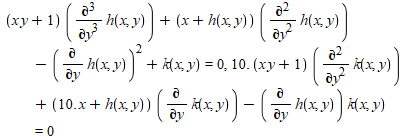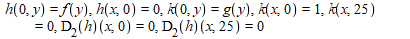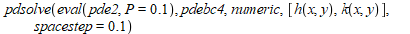## how i can pdsolve these partial differential equat...

hi

how i can pdsolve these partial differentialequations?

thanks(1)(2)(3)(4)## Problems with getting consistent execution timings...

I have been using CodeTools::Usage with Maple 17 to compare timings of a couple of matrix inversion routines against each other and against the Maple library routines LinearAlgebra::MatrixInverse and LinearAlgebra::Determinant.

The small section of code that I use to collect the cpu times for each method is:

----------------------------------------

udata := Usage(invTest(x,y,z,delta,mu), output=['cputime', 'bytesused'],
iterations=nRepeats, quiet);
dat:=udata; dat:=udata;

----------------------------------------
nRepeats has been set to 25.

In order to get the timings as accurate as possible, I only open a couple of terminal windows on my laptop so as to run the tests with as low a loaded machine as I can get. (I'm running Ubuntu 14.04 on an 8 core Intel (R) Core i7-3840QM, 2.8GHz CPU with 16Gb of memory.)

A test run consists of a sequence of test matrices each of which is run for an increasing sequence of matrix orders. I either time an implementation on its own or I time both an implementation and the Maple library routines. In the later case for each test matrix and order of matrix, I use Usage to first time the Maple routines and then the times for one of the implementations:

----------------------------------------

if compMaple then
udata := Usage(MatrixInverse(A), output=['cputime', 'bytesused'],
iterations=nRepeats, quiet);
mdat:=udata; mdat:=udata;

udata := Usage(Determinant(A), output=[cputime, bytesused],
iterations=nRepeats, quiet);
mdat:=mdat+udata;
mdat:=mdat+udata;
else
mdat[1..2] := 0:
end if:

udata := Usage(invTest(x,y,z,delta,mu), output=['cputime', 'bytesused'],
iterations=nRepeats, quiet);
dat:=udata; dat:=udata;

----------------------------------------

I have noticed the following:

a) If I time both Maple and an implementation, and the same implementation on its own I can get timings that vary up to a factor of 2 or more,

b) Changes in timings for the same test run and as close to the same environment as I can get (i.e., just the two user terminal windows open) can generate timing differences of up to 50%.

c) Sometimes `chaotic' timings are generated, for example,

n = 150, t = 3 (secs)
n = 200, t = 24 (secs)
n = 250, t = 18 (secs)

which cannot be reflecting the times required to perform the calculations. This type of behaviour always seems to occur when the reported memory bytecount is increasing from ~0.25* 10^9 to over 10^9.

Is there anything I can be doing here to get more consistent timings?

I want to use the timings from these tests in a journal article so it would be good to have the same test run under very similar conditions to return timings within a few percent difference of one another. I certainly get this sort of tolerances (<10%) if I use the Fortran cpu_time intrinsic to time Fortran code.

## What's the best way to search inserted table and r...

Using Insert->Table... and inserting information in the cells.

So I've created Table1 but I can't seem to call any row/column cell information using that name.  How do we do that?  Also, what is the best way to search for information in those cells and return information in adjacent cells?

## ImprovementsInPdsolve.mw...

Hello,

I have tryed, to run the file ImprovementsInPdsolve.mw with the Mapleversion 2017.3 but in the first example pde I got this error:

Error, (in assuming) when calling 'dsolve'. Received: 'cannot determine if this expression is true or false: not 0 <= -(1/2)*Pi'

pde,  pde and others are o.k. but pde to pde not. Can You give me a hint what's wrong?

With kindly regards

Wolfgang Gellien

## How make computations in Quantum Field Theory...

Hello all,

I would like to know how to make computations on Quantum Field Theory questions.

For example: to write Feynman diagrams for some interactions, obtain the S matrix of scattering amplitude, then deduce the diffrential cross section.

If anyone is working on these issues in the Maple community, I would be very interested.

Best regards

Jean-Luc Paillet

## differentiation of general function...

Hello people in mapleprimes

I asked a question for a similar thing before.
https://www.mapleprimes.com/questions/222751-PDEtoolsdeclare

The question I had then is not solved yet in my mind, so I think  I will ask you
again. It is about differentiation of a composite function.

with(PDEtools):
declare(f(x,y,z),g(t,y));
a:=f(x,y,z);
b:=x=g(t,y);
c:=eval(a,b);
d:=diff(c,y);
OFF;
e:=d;

h:=convert(e,diff);

For both e and d, the notation of D__1 and D__2 appear.
And, even if, with convert, I changed them to another form,
what I can get is more complicated form of h.

Is it inevitable to use D__1 and D__2 brabra in the differentiation of
composite function?

I am writing this question thinking that if there is a way of go around it,
I want to know.

And, though this is the second question, even if I use latex( ) command,
natually, codes of Tex I can get is that of the output shown on the screen, including D__1 and D__2, even though I want it to be shown with f__x and f__y or that including prime. Then, I have to edit that tex file, changing D__1 to f__x etc. which I think is very complicated modification of the text file.
I wish there is a way to modify it in Maple so as it to be direct TeX codes which  do not require me to modify.
Is it impossible to do such a thing?

diff_2.mw

## Multiplying Matrices--editing initial ...Maple will multiply two matrices for me perfectly.

However, when I change the value in one of the cells and try to re-evaluate the product, Maple starts giving me the sum of something in all of my cells.

Are you simply not allowed to ever change the initial values you set for a matrix?  This doesn't seem to make sense to me.

I have attached a screen shot to show an example with a random matrix.

## why this error message? Error, (in forget) lexical...

the following code results in an error message: Error, (in forget) lexically scoped parameter out of contextIf I click on this error message, it brings me to a page which I visited too often.

if I uncomment the irrelevant minimize command, the error message disappears.How can I prevent this error without giving irrelevant commands?

kind regards,

Harry Garst

## "unable to store %1 when datatype=%2", -9.65986559...

hello...how i can remove this error?

thanks(1)(2)## Error, (in pdsolve/numeric/par_hyp) Incorrect numb...

The following is the PDE I need to solve.

(x*y+1)*(diff(h(x, y), y, y, y))+(x+h(x, y))*(diff(h(x, y), y, y))-(diff(h(x, y), y))^2+k(x, y) = 0, (10.*(x*y+1))*(diff(k(x, y), y,y))+(10.*x+h(x,y))*(diff(k(x, y), y))-(diff(h(x, y), y))*k(x, y) = 0This is the original boundary condition:

h(0, y) = f(y), h(x, 0) = 0, k(0, y) = g(y), k(x, 0) = 1, k(x, 25) = 0, (D(h))(x, 0) = 0, (D(h))(x, 25) = 0After using pdsolve it come out the error:

pdsolve(eval(pde2, P = .1), pdebc4, numeric, [h(x, y), k(x, y)], spacestep = .1)Error, (in pdsolve/numeric/par_hyp) Incorrect number of initial conditions, expected 0, got 2

If I remove one of the boundary condition when x=0, maybe h(0,y)=f(y), then the error will be this:

Error, (in pdsolve/numeric/par_hyp) Incorrect number of initial conditions, expected 0, got 1

However if I remove both when x=0, it come out this error:

Error, (in pdsolve/numeric) initial/boundary conditions must be defined at one or two points for each independent variable

May I know what is the problem of this equations?

P/S: I know its only differentiate with respect to y and is consider to be an ODE( I need more explantion on this please) and I'm still new to maple. Thanks!!

## Use of Maple Player...

I downloaded Maple Player, and I don't know how can I use it resolve Maths problems. Kindly, could you explain to me the procedure I shall follow for the reason to use it?

## How to write to a file in MAPLE PLAYER?...

I have been unable to successfully open a text file for writing in MAPLEPLAYER, whether it is requested in the startup code section or via a document tools button.  Is there a way to write to a file in MAPLEPLAYER?  It returns a "no write access" statement.

The write works perfectly in MAPLE 2015.1

MRB

## How to use my module in Maple Player ?...

I have created and saved a MAPLE module in an .mla archive. The module contains three procedures A, B, C, where

A calls, B and C.

Once the module library has been loaded, A acccepts inputs and generates outputs.

Is it possible to create a MAPLE player worksheet which calls the module and share it with a Maple Player (only) user, so that they can then supply the inputs and observe the outputs from A using the Maple Player programme components?

Can anyone help?

MRB

## MaplePlayer App...

I have downloaded MaplePlayer on my iPad.   I see that it comes with a number of "pre-recorded" programs that it can run.  Is there a way to have it run a Maple worksheet that I have written?   If so, how do I use this app to open an existing worksheet?

Also, are there any plans for this app on the Android platform any time soon?